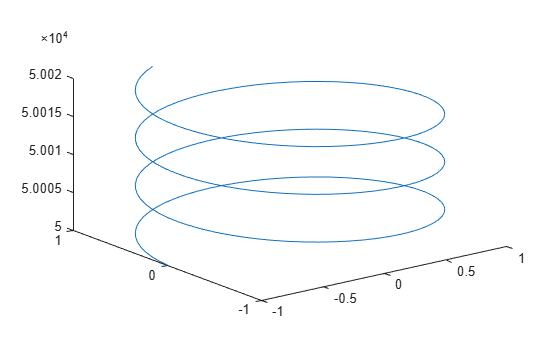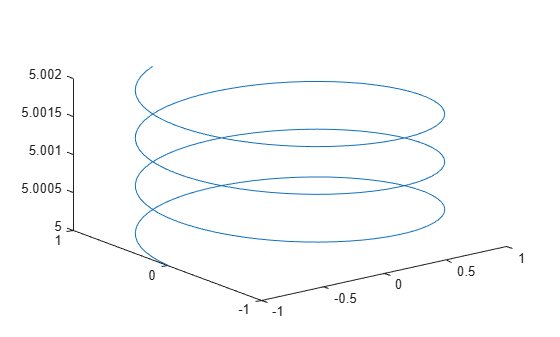# zsecondarylabel

Set z-axis secondary label

Since R2023b

## Syntax

``zsecondarylabel(label)``
``zsecondarylabel(Visible="off")``
``zsecondarylabel(Visible="on")``
``zsecondarylabel("auto")``
``zsecondarylabel(ax,___)``

## Description

example

````zsecondarylabel(label)` displays the specified text label as a secondary label on the z-axis of the current axes. If the z-axis already has a secondary label, the specified label replaces it.```

example

````zsecondarylabel(Visible="off")` hides the secondary label. You can specify the value of `Visible` as `false` or `0` to produce the same result. For example, `zsecondarylabel(Visible=0)` also hides the label.```
````zsecondarylabel(Visible="on")` displays the secondary label. You can specify the value of `Visible` as `true` or `1` to produce the same result. For example, `zsecondarylabel(Visible=true)` also displays the label. This syntax has no effect when the label is empty.```
````zsecondarylabel("auto")` resets the secondary label to its automatic state, which displays a secondary label in some cases. For example, a secondary label might automatically appear when you plot datetime values or very large numbers.```

example

````zsecondarylabel(ax,___)` modifies the secondary label for the target axes. Specify the target axes before any of the input argument combinations in the previous syntaxes.```

## Examples

collapse all

Plot the `membrane` data set as a surface. Then add a secondary label, `"x100 m"`.

```Z = membrane; surf(Z) zsecondarylabel("x100 m")```Create three vectors and plot them.

```z = 50000:0.1:50020; x = sin(z); y = cos(z); plot3(x,y,z)```Hide the secondary label on the z-axis. The exponent label disappears.

`zsecondarylabel(Visible="off")`Create two surface plots in a tiled chart layout. When you call the `nexttile` function, specify an output argument to get the axes object for later use.

```tiledlayout(1,2,Padding="tight") Z = membrane; % First plot ax1 = nexttile; surf(Z) % Second plot ax2 = nexttile; surf(Z)```Add a secondary label, `"x100 m"`, to the z-axes of both plots by specifying the array `[ax1 ax2]` as the first argument to the `zsecondarylabel` function.

`zsecondarylabel([ax1 ax2],"x100 m")`## Input Arguments

collapse all

Label text, specified as a string scalar, string vector, character vector, or cell array of character vectors. To display one line of text, specify a string scalar or character vector. To specify multiple lines of text, specify a string vector or cell array of character vectors.

Example: `zsecondarylabel("Hurray for me!")`

Example: `zsecondarylabel('Hurray for you!')`

Target axes, specified as an `Axes` object or an array of `Axes` objects.

If you do not specify the target axes, then `zsecondarylabel` uses the current axes.

## Version History

Introduced in R2023b